# 字符提取，其实很简单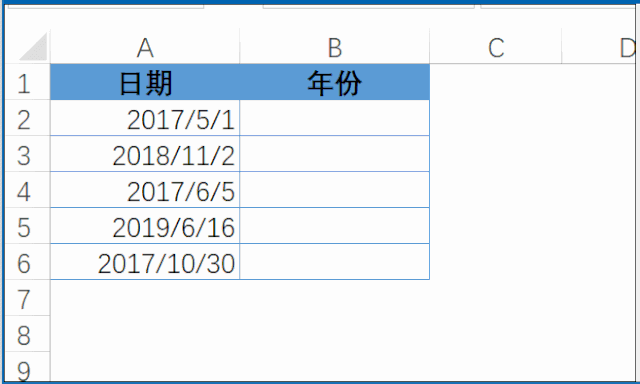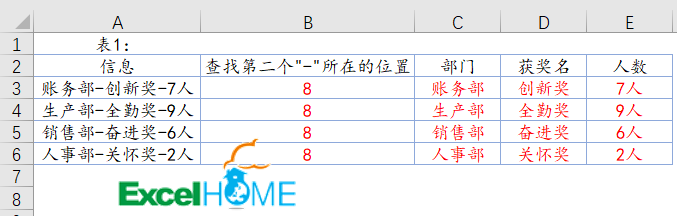LEFT函数：

=LEFT(字符串,[提取几个字符])

=LEFT(A3,3)

RIGHT函数：

=RIGHT(字符串,[提取几个字符])

=RIGHT(A3,2)

MID函数：

=MID(字符串,开始提取的位置,提取几个字符)

=MID(A3,5,3)

BASE函数：

=BASE(数字,基数,[字符长度])

=BASE(678,10,4)

FIND函数：

=FIND(要查找的字符,被查找的字符,[从第几个字符开始查找])

=FIND(“-“,A3,5)

1：根据表2身份证号码提取出生年月并生成称呼(性别为女，称女士；性别为男，称先生)。
1）出生日期，C3单元格自定义单元格格式为：####”年”##”月”##”日”，然后输入公式向下填充，
=–MID(B3,7,8)

2）称呼：D3单元格输入以下公式并向下填充：
=LEFT(A3)&IF(-1^MID(B3,17,1)+1,”女士”,”先生”)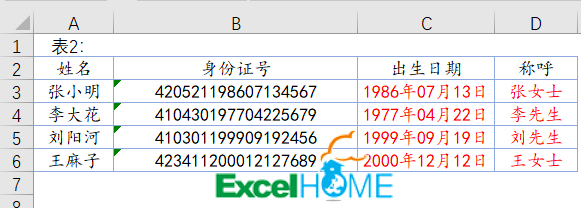2、根据表3产品信息提取尺码。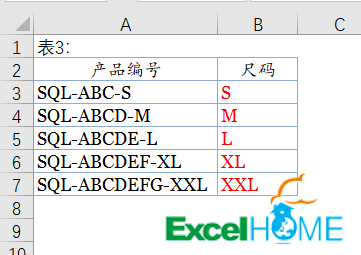B3单元格输入公式，再向下填充。
=MID(A3,FIND(“-“,A3,FIND(“-“,A3)+1)+1,4)

3、提取表4中的数字。B3单元格输入公式，Ctrl+Shift+Enter三键回车，再向下填充。
=MAX(IFERROR(–LEFT(A3,ROW(\$1:\$10)),))

B3单元格输入公式，Ctrl+Shift+Enter三键回车，再向下填充。
=MAX(IFERROR(–MID(A3,ROW(\$1:\$9),{1,2,3,4}),))

4、根据表5中A列编号修正为统一格式。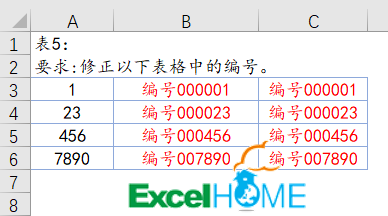B3单元格输入以下公式，再向下填充。

=”编号”&BASE(A3,10,6)

=”编号”&RIGHT(“00000″&A3,6)

5、计算表6中各部门的人数。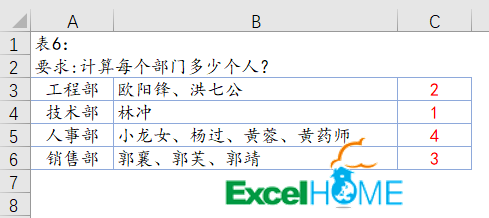C3单元格输入公式，Ctrl+Shift+Enter三键回车。
=SUM(N(MID(B3,ROW(\$1:\$50),1)=”、”))+1

6、提取表7中的姓名及手机号。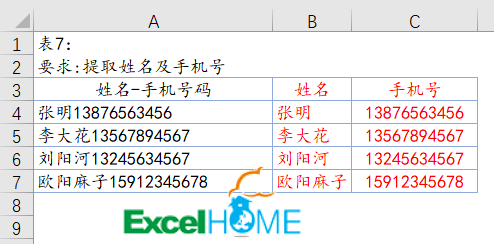=LEFT(A4,LENB(A4)-LEN(A4))

=RIGHT(A4,LEN(A4)*2-LENB(A4))

https://pan.baidu.com/s/1diDYm9aqLl52haR-SAZ6eA